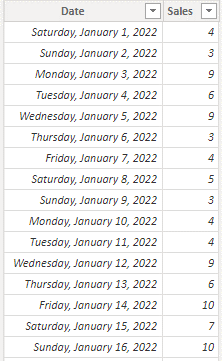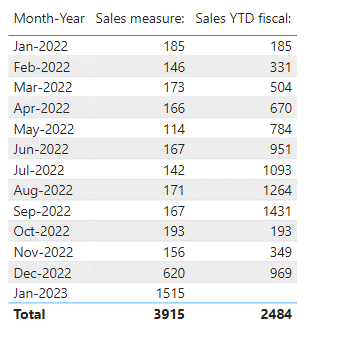cancel
Showing results for
Did you mean:Helper I

## Fiscal YTD through Last Month

I have a measure that was working until we rolled into 2023.  The desired output is fiscal YTD sales through the prior month.  For example, we are in January now, so the output would show Fiscal YTD thorugh the end of December.

Here is the formula I'm using:

Sales YTD Last Month =
CALCULATE(SUM(
'All Sales'[Amount]),
DATESYTD('REF Dates'[Date], "9/30"),
MONTH('REF Dates'[Date]) < MONTH(TODAY())
)

Prior to Jan1, it gave the desired results.  Now it gives no results.  I should note that I have tried replacing the [Date] field about with the [Fiscal Date Ref] and still did not receive an output.

REF Dates is a date table:All Sales is my table with sales data:1 ACCEPTED SOLUTIONCommunity Support

Hi @charbaugh ,

According to your description, here are my steps you can follow as a solution.

(1) This is my test data.

Calendar:

``````Calendar =
CALENDAR ( DATE ( 2021, 7, 1 ), DATE ( 2023, 6, 30 ) ),
"Month-Year", FORMAT ( [Date], "mmm-yyyy" ),
"Month-Year sort", EOMONTH ( [Date], 0 )
)``````

Sales:...(2) We can create a measure.

``````Sales YTD fiscal: =
VAR _currentmonthend =
EOMONTH ( MAX ( 'Calendar'[Date] ), 0 )
VAR _currentmonthendinfo =
MAX ( Sales[Date] )
RETURN
IF (
HASONEVALUE ( 'Calendar'[Month-Year] ),
IF (
_currentmonthend = _currentmonthendinfo,
CALCULATE ( SUM(Sales[Sales]), DATESYTD ( 'Calendar'[Date], "9-30" ) )
),
CALCULATE (  SUM(Sales[Sales]), DATESYTD ( 'Calendar'[Date], "9-30" ) )
)``````

(3) Then the result is as follows.If the above one can't help you get the desired result, please provide some sample data in your tables (exclude sensitive data) with Text format and your expected result with backend logic and special examples. It is better if you can share a simplified pbix file. Thank you.

Best Regards,

Neeko Tang

If this post  helps, then please consider Accept it as the solution  to help the other members find it more quickly.

2 REPLIES 2Community Support

Hi @charbaugh ,

According to your description, here are my steps you can follow as a solution.

(1) This is my test data.

Calendar:

``````Calendar =
CALENDAR ( DATE ( 2021, 7, 1 ), DATE ( 2023, 6, 30 ) ),
"Month-Year", FORMAT ( [Date], "mmm-yyyy" ),
"Month-Year sort", EOMONTH ( [Date], 0 )
)``````

Sales:...(2) We can create a measure.

``````Sales YTD fiscal: =
VAR _currentmonthend =
EOMONTH ( MAX ( 'Calendar'[Date] ), 0 )
VAR _currentmonthendinfo =
MAX ( Sales[Date] )
RETURN
IF (
HASONEVALUE ( 'Calendar'[Month-Year] ),
IF (
_currentmonthend = _currentmonthendinfo,
CALCULATE ( SUM(Sales[Sales]), DATESYTD ( 'Calendar'[Date], "9-30" ) )
),
CALCULATE (  SUM(Sales[Sales]), DATESYTD ( 'Calendar'[Date], "9-30" ) )
)``````

(3) Then the result is as follows.If the above one can't help you get the desired result, please provide some sample data in your tables (exclude sensitive data) with Text format and your expected result with backend logic and special examples. It is better if you can share a simplified pbix file. Thank you.

Best Regards,

Neeko Tang

If this post  helps, then please consider Accept it as the solution  to help the other members find it more quickly.Helper I

This appears to give me a good fiscal YTD, all the way up thorugh today.  But what I need is fiscal YTD, up to the end of last fiscal month.  I'm trying to figure out how to build a simplified dataset to share, but this is part of a very large and complex file.